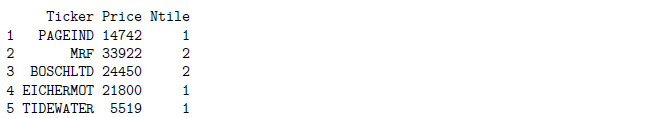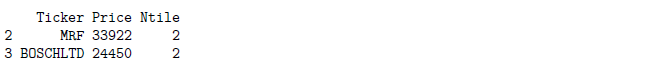# R Weekly Bulletin Vol – XThis week’s R bulletin will cover topics on grouping data using ntile function, how to open files automatically, and formatting an Excel sheet using R. Hope you like this R weekly bulletin. Enjoy reading!

### Shortcut Keys

1. Fold selected chunk - Alt+L
2. Unfold selected chunk - Shift+Alt+L
3. Fold all - Alt+0

### Problem Solving Ideas

#### Grouping data using ntile function

The ntile function is part of the dplyr package, and is used for grouping data. The syntax for the function is given by:

ntile(x, n)

Where, “x” is the vector of values and “n” is the number of buckets/groups to divide the data into.

Example:

In this example, we first create a data frame from two vectors, one comprising of Stock symbols, and the other comprising of their respective prices. We then group the values in Price column in 2 groups, and the ranks are populated in a new column called “Ntile”. In the last line we are selecting only those values which fall in the 2nd bucket using the subset function.

library(dplyr)
Ticker = c("PAGEIND", "MRF", "BOSCHLTD", "EICHERMOT", "TIDEWATER")
Price = c(14742, 33922, 24450, 21800, 5519)

data = data.frame(Ticker, Price)

data$Ntile = ntile(data$Price, 2)
print(data)ranked_data = subset(data, subset = (Ntile == 2))
print(ranked_data)#### Automatically open the saved files

If you are saving the output returned upon executing an R script, and also want to open the file post running the code, one can you use the shell.exec function. This function opens the specified file using the application specified in the Windows file associations.

A file association associates a file with an application capable of opening that file. More commonly, a file association associates a class of files (usually determined by their filename extension, such as .txt) with a corresponding application (such as a text editor).

The example below illustrates the usage of the function. shell.exec(filename)

Example:

df = data.frame(Symbols=c("ABAN","BPCL","IOC"),Price=c(212,579,538))
write.csv(df,"Stocks List.csv")
shell.exec("Stocks List.csv")

#### Quick format of the excel sheet for column width

We can format the excel sheets for column width using the command lines given below. In the example, the first line will load the excel workbook specified by the file name. In the third & the fourth line, the autoSizeColumn function adjusts the width of the columns, which are specified in the “colIndex”, for each of the worksheets. The last line will save the workbook again after making the necessary formatting changes.

Example:

wb = loadWorkbook(file_name)
sheets = getSheets(wb)
autoSizeColumn(sheets[], colIndex=1:7)
autoSizeColumn(sheets[], colIndex=1:5)
saveWorkbook(wb,file_name)

### Functions Demystified

#### choose function

The choose function computes the combination nCr. The syntax for the function is given as:

choose(n,r)

where, n is the number of elements r is the number of subset elements

nCr = n!/(r! * (n-r)!)

Examples:

choose(5, 2)


 10

choose(2, 1)


 2

#### sample function

The sample function randomly selects n items from a given vector. The samples are selected without replacement, which means that the function will not select the same item twice. The syntax for the function is given as:

sample(vector, n)

Example: Consider a vector consisting of yearly revenue growth data for a stock. We select 5 years revenue growth at random using the sample function.

Revenue = c(12, 10.5, 11, 9, 10.75, 11.25, 12.1, 10.5, 9.5, 11.45)
sample(Revenue, 5)

 11.45 12.00 9.50 12.10 10.50

Some statistical processes require sampling with replacement, in such cases you can specify replace= TRUE to the sample function.

Example:

x = c(1, 3, 5, 7)
sample(x, 7, replace = TRUE)

 7 1 5 3 7 3 5

#### runif and rnorm functions

The runif function generates a uniform random number between 0 and 1. The argument of runif function is the number of random values to be generated.

Example:

# This will generate 7 uniform random number between 0 and 1.
runif(7)

 0.6989614 0.5750565 0.6918520 0.3442109 0.5469400 0.7955652 0.5258890

# This will generate 5 uniform random number between 2 and 4.
runif(5, min = 2, max = 4)

 2.899836 2.418774 2.906082 3.728974 2.720633

The rnorm function generates random numbers from normal distribution. The function rnorm stands for the Normal distribution’s random number generator. The syntax for the function is given as:

rnorm(n, mean, sd)

Example:

# generates 6 numbers from a normal distribution with a mean of 3 and standard deviation of 0.25
rnorm(6, 3, 0.25)

 3.588193 3.095924 3.240684 3.061176 2.905392 2.891183

### Next Step

We hope you liked this bulletin. In the next weekly bulletin, we will list more interesting ways and methods plus R functions for our readers.